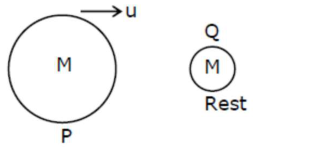# Given below are two statements :Question:

Given below are two statements : one is labelled as Assertion A and the other is labelled as Reason R.

Assertion A : Body 'P' having mass M moving with speed 'u' has head-on collision elastically with another body ' $Q$ ' having mass ' $m$ ' initially at rest. If $\$ m<$Reason$R$: During elastic collision, the momentum and kinetic energy are both conserved. In the light of the above statements, choose the most appropriate answer from the options given below: 1. (1)$\mathrm{A}$is correct but$\mathrm{R}$is not correct. 2. (2) Both$\mathrm{A}$and$\mathrm{R}$are correct but$\mathrm{R}$is NOT the correct explanation of$\mathrm{A}$. 3. (3)$\mathrm{A}$is not correct but$\mathrm{R}$is correct. 4. (4) Both$\mathrm{A}$and$\mathrm{R}$are correct and$\mathrm{R}$is the correct explanation of$\mathrm{A}$. Correct Option: 4, Solution: (4)$m<

$e=\frac{V_{2}-V_{1}}{u_{1}-u_{2}}$

For elastic collision $\rightarrow \mathrm{e}=1$

$1=\frac{v_{2}-u}{u-0}$

$\mathrm{u}=\mathrm{v}_{2}-\mathrm{u}$

$v_{2}=2 u$

In elastic collision kinetic energy \& momentum are conserved.